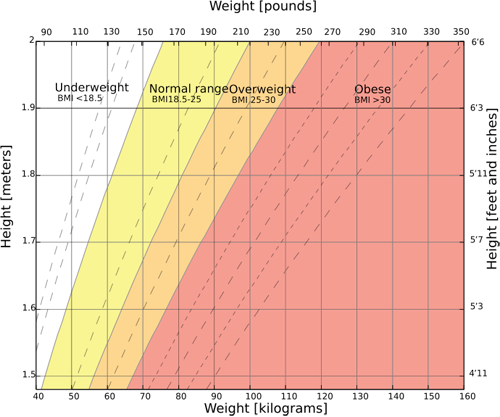# Ideal Weight Calculator

Please select the gender, enter the height and weight of the person in centimeters and in kilogram. Then click on the calculate button to calculate his/her ideal weight.

Ideal Weight Calculation Form
1. Female Male
2. cm
3. kg

## What is an ideal weight?

According to doctors an ideal weight would be inside the healthy weight range for women as defined by the Body Mass Index - i.e. a BMI between 19 and 24.9. (See Figure below)## How to calculate ideal weight for men and women?

Ideal weight for both men and women is calculated using a formula given below. The formula also gives you a rate between height and weight.

• Ideal weight for women: 45.5 + 2.3 X (height in inches - 60)
• Ideal weight for men: 50 + 2.3 X (height in inches - 60)